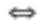Chapter 8.3, Problem 19ES### Discrete Mathematics With Applicat...

5th Edition
EPP + 1 other
ISBN: 9781337694193

#### Solutions

Chapter
Section### Discrete Mathematics With Applicat...

5th Edition
EPP + 1 other
ISBN: 9781337694193
Textbook Problem
1 views

# In 19-31, (1) prove that the relation is an equivalence relation, and (2) describe the distinct equivalence classes of each relation. 19. A is the set of all students at your college. R is the relation defined on A as follows: For everyx and y in A. xRyx has the same major (or double major) as y.(Assume “undeclared” is a major.) S is the relation defined on A as follows: For every x , y , ∈ A , xsyx is the same age as y.

To determine

(a)

To prove:

(1) The given relation is an equivalence relation,

(2) Describe the distinct equivalence classes of each relation.

Explanation

Given information:

A is the set of all students at your college.

R is the relation defined on A as follows: For all x and y in A ,

x R y ⇔ x has the same major (or double major) as y.

(Assume “undeclared” is a major.)

Proof:

(1). A relation will be equivalence relation if it is reflexive, symmetric and transitive.

Reflexive:

Since an individual always has the same major as itself, (x,x)R for all xA and thus R is indeed reflexive.

Symmetric:

Let us assume that (x,y)R.

By the definition of R: x and y have the same major.

But then y and x also have the same major:

(y,x)R

Since (x,y)R and (y,x)R, R is symmetric.

Transitive:

Let us assume that (x,y)R and (y,z)R

To determine

(b)

To prove:

(1) The given relation is an equivalence relation,

(2) Describe the distinct equivalence classes of each relation.

### Still sussing out bartleby?

Check out a sample textbook solution.

See a sample solution

#### The Solution to Your Study Problems

Bartleby provides explanations to thousands of textbook problems written by our experts, many with advanced degrees!

Get Started